# RD Sharma Solutions Class 9 Chapter 2 Exponents of Real Number

Read RD Sharma Solutions Class 9 Chapter 2 Exponents of Real Number below, students should study RD Sharma class 9 Mathematics available on Studiestoday.com with solved questions and answers. These chapter wise answers for class 9 Mathematics have been prepared by teacher of Grade 9. These RD Sharma class 9 Solutions have been designed as per the latest NCERT syllabus for class 9 and if practiced thoroughly can help you to score good marks in standard 9 Mathematics class tests and examinations

Exercise 2.1

Question 1. Simplify the following:-
(i) 3(a4b3)10 × 5(a2b2)3
(ii) (2x -2 y3)3
(iii) ((4 × 107 )  (6 × 10-5))/(8 × 104
(iv) (4ab2  (-5ab3 ))/(10a2 b2 )
(v) {(x2 y2)/(a2 b2 )}n
(vi) (a3n-9)6/a2n-4
Solution 1.

(i) We have 3(a4b3)10 × 5(a2b2)3

By using identity:-  (xm)n = xmn

After simplification we get

= 3(a40b30) × 5(a6b6)

= 3 × a40 × b30 × 5 × a6 × b6

By using identity:-  xm × xn = xm+n

= 15 × a46 × b36

(ii) (2x -2 y3)3

By using identity:- (x-m)= 1/x and (xm)n = xmn

After simplification we get

= 23 × 1/x2x3 × y3×3

= 8 × 1/x6 × y9

= 8  y9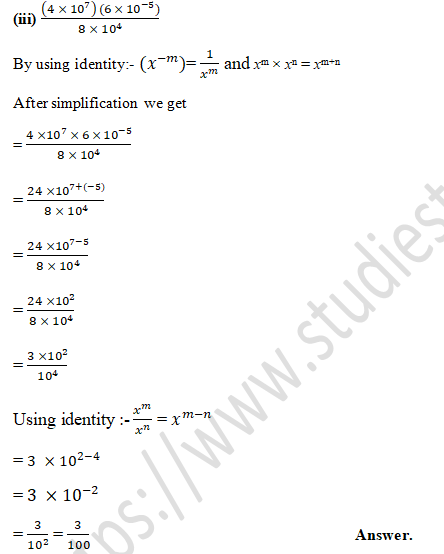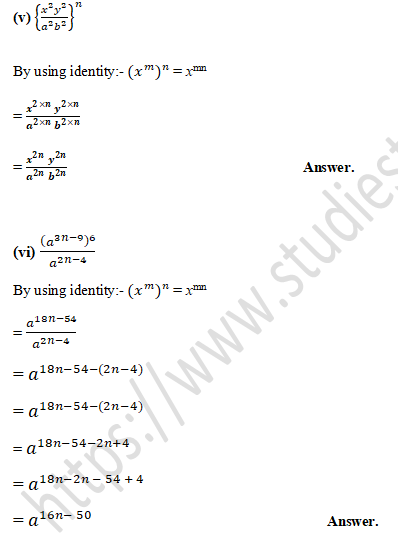Question 2. If a = 3 and b = - 2, find the value of:
(i) aa + bb
(ii) ab + ba
(iii) (a+b)ab
Solution 2.
(i) We have  aa + bb
Put the value a = 3 and b = -2 in equation given above
= 33 + (-2)-2
= 3 × 3 × 3 + 1/((-22))
= 27 +1/((-2 ×-2))
= 27 +1/4
= 27/1+1/4
Take the LCM of denominators 1 and 4 = 4
= (108+1)/4

(ii) We have  ab + ba
Put the value a = 3 and b = –2 in equation given above
= 3-2 + (–2)3
= 1/((32)) + (–2 × –2 × –2)
= 1/9 – 8
= 1/9-8/1
Take the LCM of denominators 9 and 1 = 9
= (1-72)/9

(iii) We have (a+b)ab
Put the value a = 3 and b = –2 in equation given above
= {3 + (–2)}3×(–2)
= {3 + (–2)}–6
= {3 – 2}–6
= (1) –6
= 1/16

Question 3.  Prove that: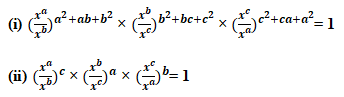Solution 3.Question 4. Prove that: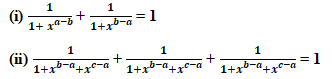Solution 4.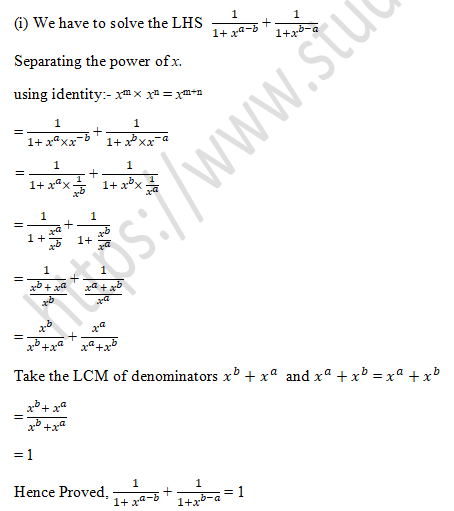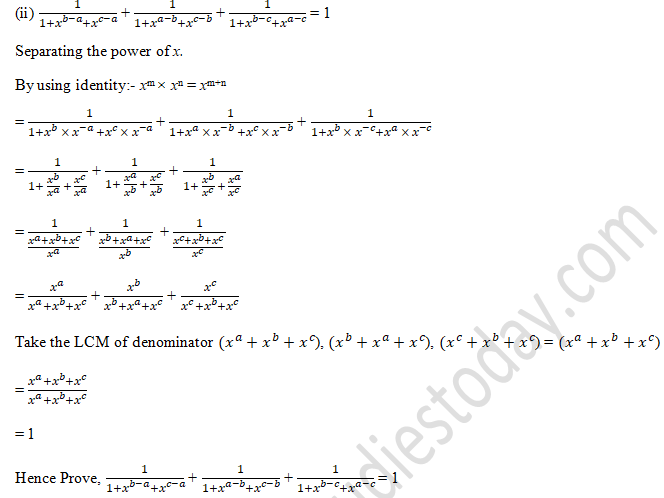Question 5. Prove that: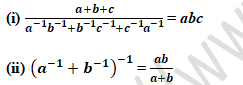Solution 5.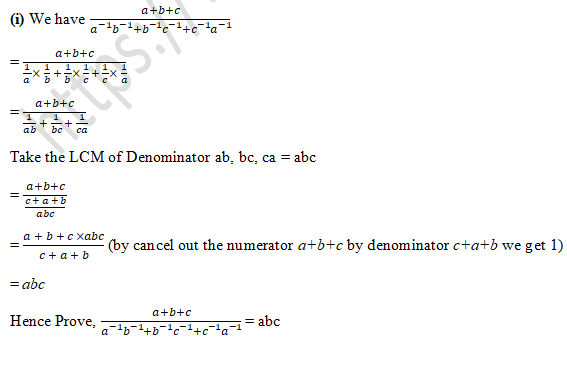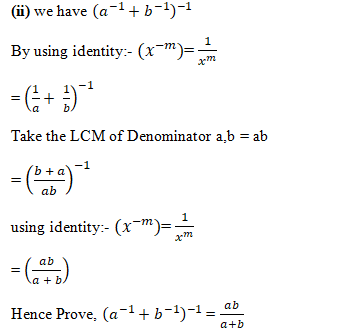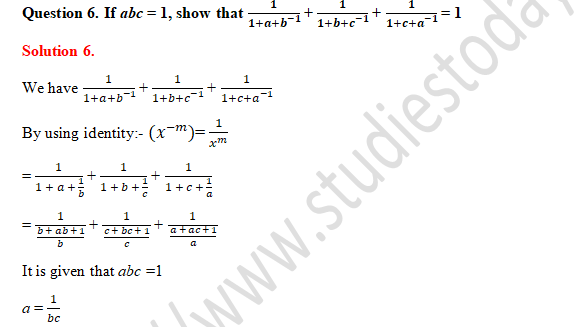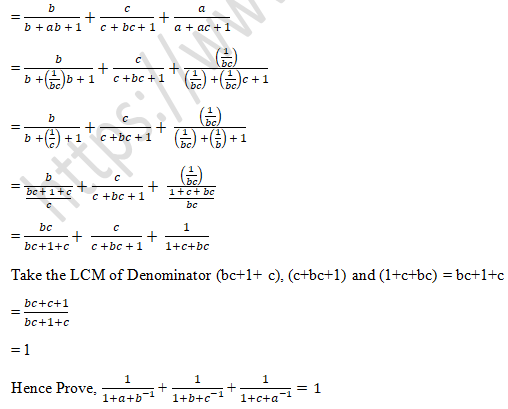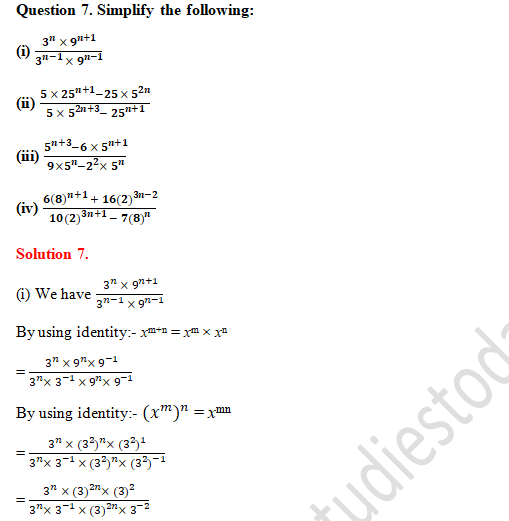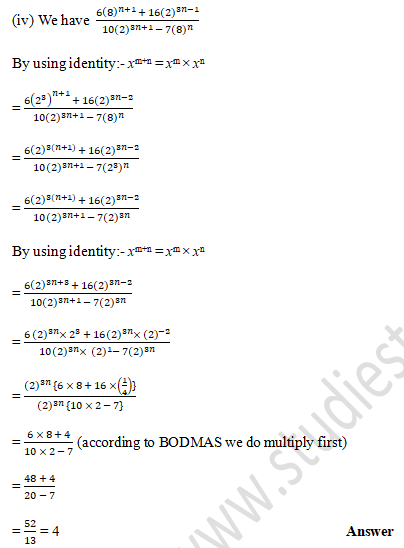Question 8. Solve the following equation for x:
(i) 7(2x+3) = 1
(ii) 2(x+1)= 4(x-3)
(iii) 2(5x+3) = 8(x+3)
(iv) 42x = 1/32
(v) 4(x-1)×(0.5)(3-2x) = (1/8)x
(vi) 2(3x-7) = 256
Solution 8.
(i) we have 7(2x+3) = 1
By using identity:- x0 =1
7(2x+3) = 70
We should calculate the value of x through their powers.
2x + 3 = 0
2x = -3

(ii) we have 2(x+1)= 4(x-3)
By using identity:- (xm)n = xmn
2(x+1)= (22)(x-3)
2(x+1)= (2)2(x-3)
2(x+1) = (2)(2x-6)
We should calculate the value of x through their powers.
x + 1 = 2x – 6
x – 2x = – 6 – 1
– x = – 7

(iii) we have 2(5x+3) = 8(x+3)
By using identity:- (xm)n = xmn
2(5x+3) = (23)(x+3)
2(5x+3)=(2)3(x+3)
2(5x+3)=2(3x+9)
We should calculate the value of x through their powers.
5x+3 = 3x+9
5x – 3x = 9 – 3
2x = 6
x = 6/2

(iv) we have 42x = 1/32
(4)2x = 1/(2)
(22)2x = 1/(2)
By using identity:- (xm)n = xmn
(2)4x = 2(-5)
We should calculate the value of x through their powers.
4x = -5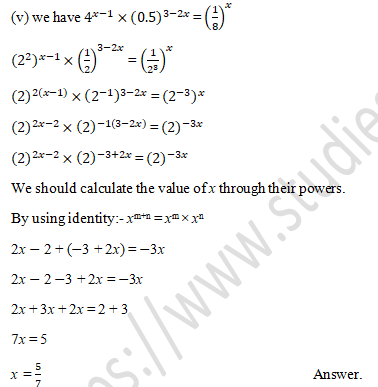(vi) 2(3x-7) = 256
We should calculate the value of x through their powers.
2(3x-7) = 28
3x - 7 = 8
3x = 8 + 7
3x = 15
x = 15/3

Question 9.  Solve the following equations for x:
(i) 22x -22x+3+24 = 0
(ii) 3(2x+4)+1=2×3(x+2)
Solution 9.Question 10. If 49392 = a4b2c3, find the value of a, b and c where a, b and c are different positive primes.
Solution 10.

It is Given that 49392 = a4b2c3
By prime factorisation method we get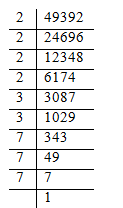By prime factorisation we can observe that 24,32 and 73.
Hence the value of a = 2, b = 3 and c = 7                                                          Answer.

Question 11. If 1176 = 2a3b7c, find a,b and c.
Solution 11
It is Given that 1176 = 2a3b7c
By prime factorisation method we get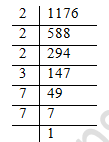By prime factorisation we can observe that 23,31 and 72.
Hence the value of a = 3, b = 1 and c = 2                                                          Answer.

Question 12.  Given 4725 = 3a 5b 7c, find
(i) the integral value of a, b and c
(ii) the value of 2-a3b7c
Solution 12.
(i) It is Given that 4725 = 3a 5b 7c
By prime factorisation method we get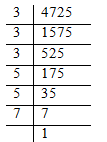By prime factorisation we can observe that 33,52 and 71.
Hence the value of a = 3, b = 2 and c = 1.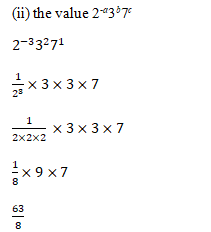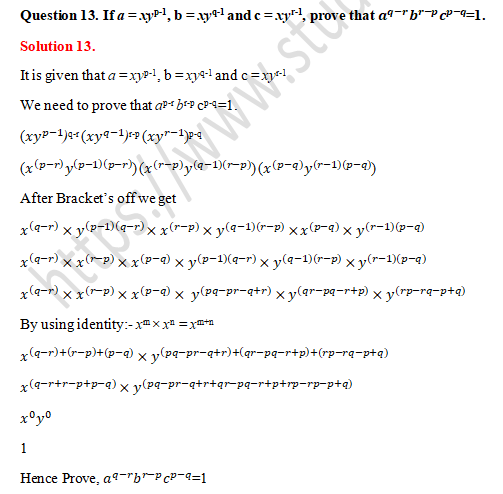Exercise 2.2

Question 1.  Assuming that x,y,z are positive real numbers, simplify each of the following:Solution 1.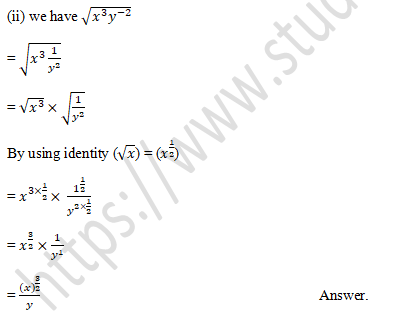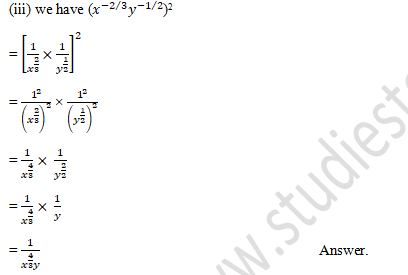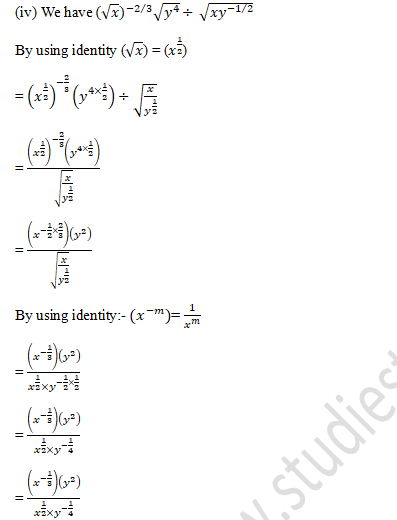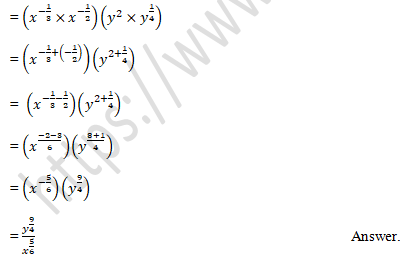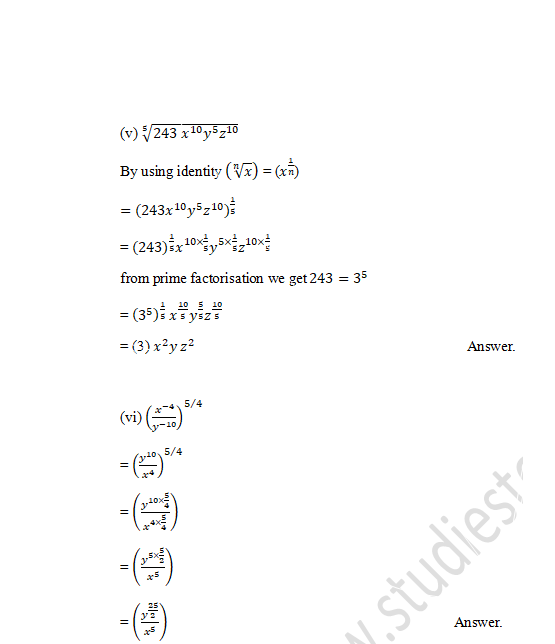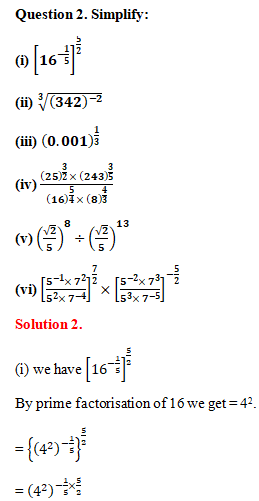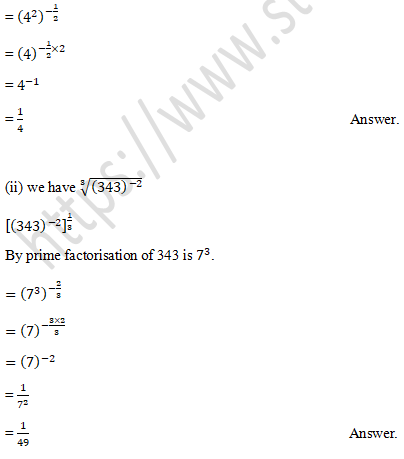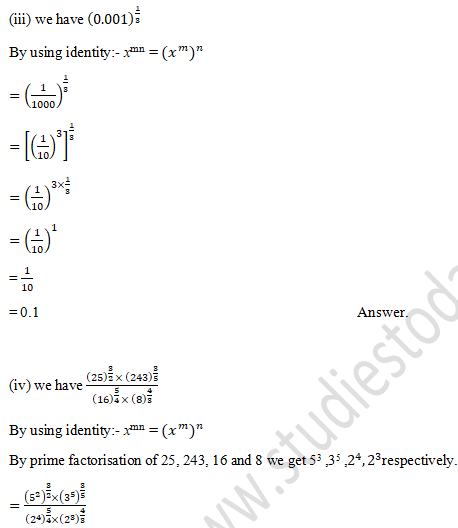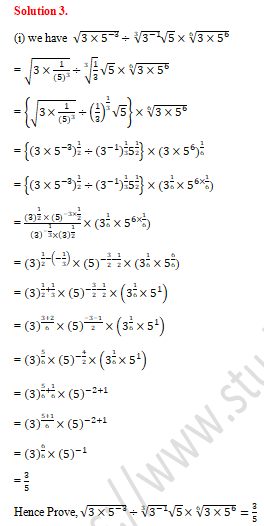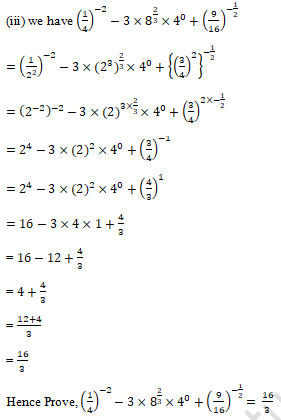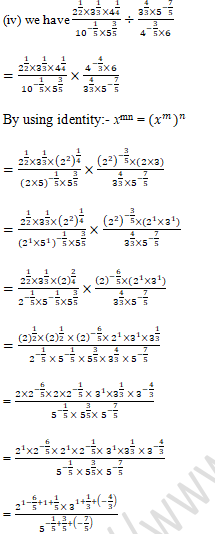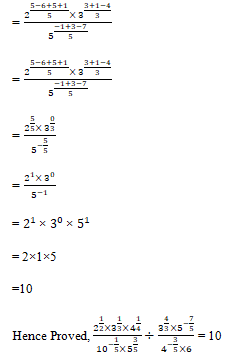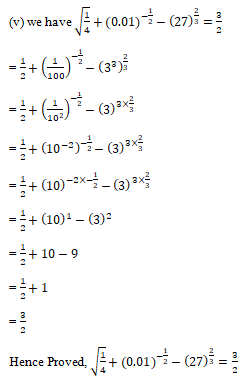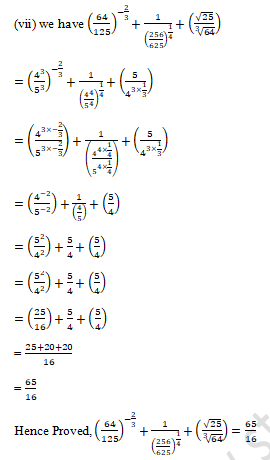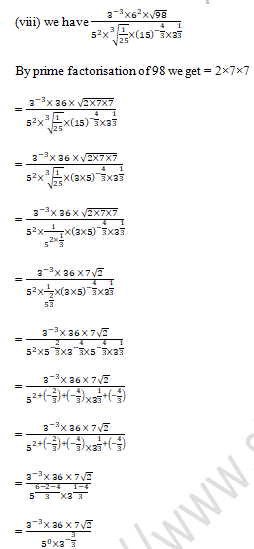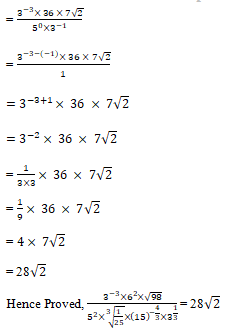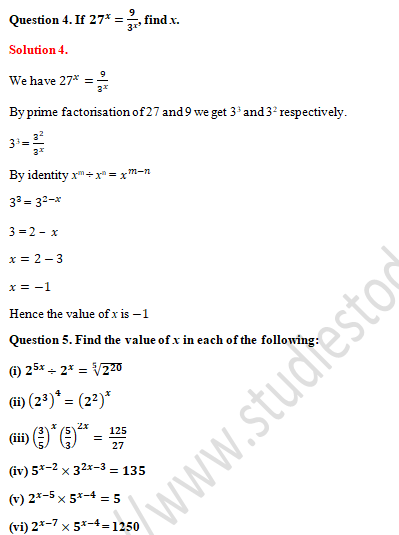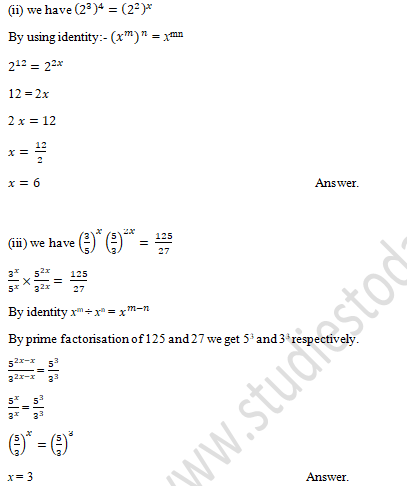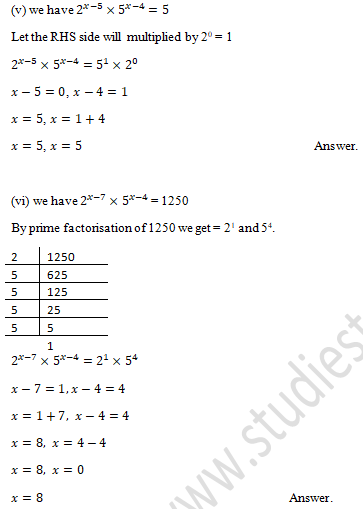Exercise 2.3
Question 1. Write (625)-1/4 in decimal form.
Solution  1.
We have (625)-1/4
By the prime factorisation 625 we get 5×5×5×5
= (54)-1/4
= 5-4/4
= 5-1
= 1/5
= 0.2

Question 2.  State the product law of exponents.
Solution  2.
The product law of exponents is, “If a is any real number and m, n are positive integers, then x× x= x(m+n)
With the reference of above definition we get,
x× x=(x×x×x……………to m factors)×(x×x×x……………to n factors)
= a×a×a……………….. to (m+n) factors
= a(m+n)
Hence, am×an = a(m+n)

Question 3. State the quotient law of exponents.
Solution 3.
In the quotient law of exponents we need to divide two powers with the same base as given in example:- xm/xn
Here a≠0.
When base is same and exponents in division we should subtracting the exponents. Here m and n are the positive integers, then xm/xn =x(m-n)

Question 4. State the power law of exponents.
Solution  4.
In the power rule of exponents we need to raise a power to a power by multiply the exponents with each other.  If a is any real number and m, n are positive integers, then
each other.  If a is any real number and m, n are positive integers, then
(xm )=xm× xm× x× xm……………..n factors
(xm )=(x×x×x×…..m)× (x×x×x×…..n)
(xm )=(x×x×x…..mn) factors
(xm )= xmn

Question 5. If 24×42=16x, then find the value of x.

Solution 5.

We have 24×4= 16x

By the prime factorisation of 16 we get 2×2×2×2.

24×42  = (24 )x

24×(22)2=(24)

2× 24=(24)x

28=24x

By equating the exponents we get

8 = 4x

8/4=x

Question 6. If 3x-1=9 and 4y+2=64, what is the value of x/y?
Solution 6.
We have 3x-1=9
By the prime factorisation of 9 we get 3×3
3x-1 = 3×3
3x-1 = 32
By equating the exponents we get
x-1=2
x=2+1
x=3

4y+2 = 64
By the prime factorisation of 64 we get 4×4×4
4y+2 = 4×4×4
4y+2 = 43
By equating the exponents we get
y+2=3
y=3-2
y=1
Therefore,
x/y=3/1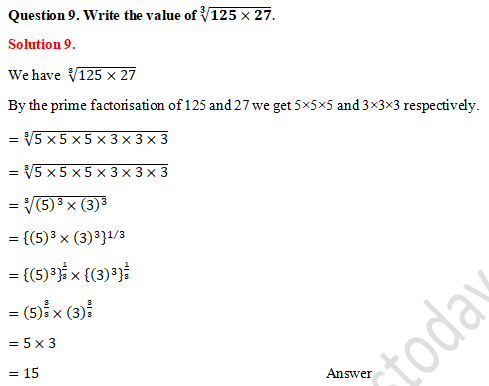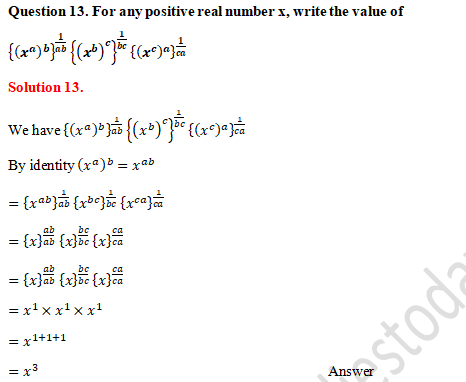Question 14. If (x-1)3= 8, What is the value of (x+1)?
Solution 14.
We have (x-1)= 8
By the prime factorisation of 8 we get 2×2×2
(x-1)= 23
Equalising the base we get
x-1=2
x=2+1
x=3
Value of x put in given equation (x+1)2
=(3+1)
=(4)2
=4×4